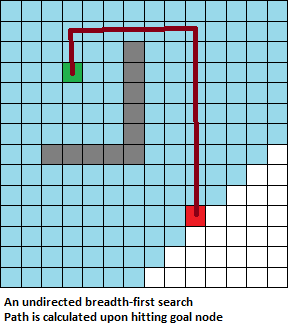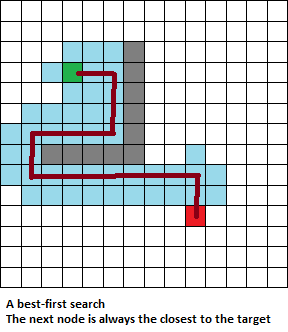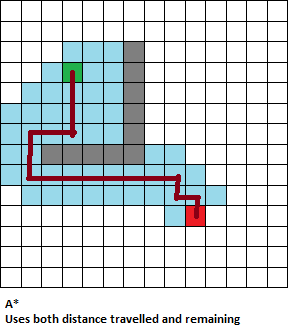## Saturday, 22 November 2014

### The Why of A*

For pathfinding, breadth-first search (BFS) is almost always preferable to depth-first search (DFS). The general problem of a breadth-first search is that it is resource-intensive. Depth-first follows a single path that grows as the path is explored. Breadth-first explores multiple paths at once, with the amount of paths growing exponentially.Both DFS and BFS are brute force attempts at finding a path, but they express themselves in different ways. A depth-first will in all likelihood find a path that won’t directly go from A to B, but follow the rules which govern how to choose the next position to explore. A breadth-first search might find a more direct path from A to B, but the result will take much more time to compute – something that’s unforgivable in a game which requires immediate feedback.

There are ways of directing a BFS so that it doesn’t take as much computational power. A* is the best of these, but it’s worth at least glancing over why this is the case. A* works on the following equation:
f = g + h
Where g is the total distance travelled, h is the estimated distance left to travel, and the smallest f is chosen next. An undirected BFS would be the equivalent of ignoring h altogether, so it’s worth exploring the role of h.

One strategy with a BFS is to just take into account the shortest distance to the goal. So whatever node is “closest” (and there are various strategies for working this out) should be the next node to search. This strategy works well if there are no obstacles directly between the start node and the goal node, as the closest node will always bring the path closer to the goal. The strategy fails, however, if there are obstacles, because the path will inevitably explore those dead ends like a DFS would.A* gets around this problem by also taking into account just how far the path has travelled. In other words, what makes a path the most fruitful to explore is the estimated total of the journey. A path that goes down a dead end will have an overall larger journey than a path that took a deviation before the dead end path.With the A* algorithm, the final output would be a path that always has the least number of moves between A and B calculated with exploring as little of the search tree as necessary. The algorithm, like with any algorithm, has its limitations, but ought to be used where the start and end goals are known and need to be computed.